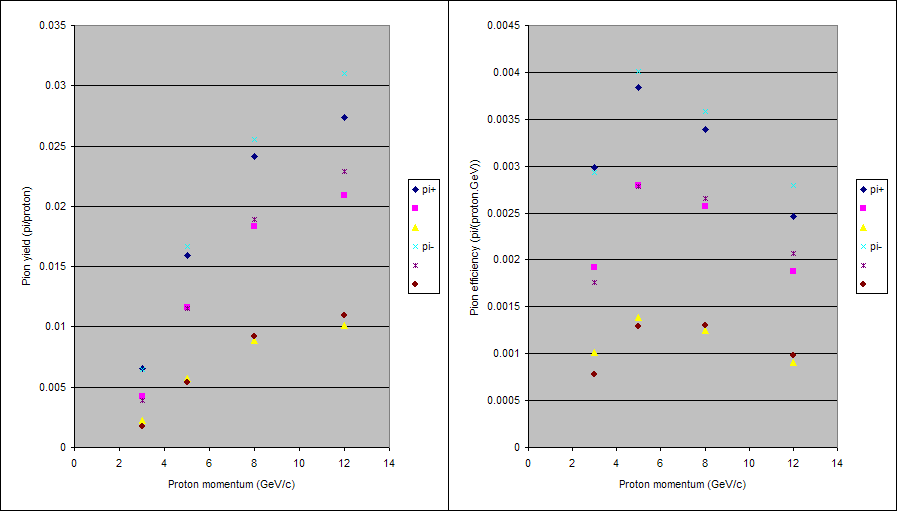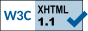# Comparison of HARP tantalum 5% interaction length data against simulations using MARS 15.07

Stephen Brooks (s.j.brooks@rl.ac.uk)   August 2007

See the HARP publication http://arxiv.org/abs/0706.1600, the goal is to replicate figure 30 in a simulation.

The graph shows yield of π+ and π integrated over three different regions:

• the full acceptance of the large-angle spectrometer (LAS), 100 MeV/c < p < 700 MeV/c and 0.35 < θ < 1.55 (radians);
• a restricted angle range, the above with θ < 0.95;
• a range further restricted in momentum, 0.35 < θ < 0.95 and 250 MeV/c < p < 500 MeV/c.

Incident proton momenta used in the graph are 3, 5, 8 and 12 GeV/c.

Simulations were made in MARS for each of these cases with at least 1 million incident protons and the yield of pions integrated over the above ranges.  Incoming beam was assumed to be a pencil beam (no spatial or angular spread) with a 1% RMS Gaussian spread in momentum around the nominal value.

Dimensions for the 5% interaction length tantalum target were taken from http://pppa.group.shef.ac.uk/harp/ta5.html.  The simulations used a cylinder of length 5.6 mm (nominal) and radius 15.07063 mm (average of measured values).

 Table 1.  Integrals of pion yield in the three regions.
Proton momentum (GeV/c)Incident protons simulatedπ+ per protonπ per proton
33.453 × 1060.0065890.004240.0022440.0064660.0038750.001728
51 × 1060.0159150.0115910.0057590.0166320.0115570.005354
81 × 1060.0241460.0183040.00890.0255170.0189290.009239
121 × 1060.0273870.0208830.0101010.0309780.0229040.01094

Note that the three integration regions are listed left-to-right in decreasing order of size in these tables.  In figure 1 it should be clear which are which, as with the graph it is based on.

 Table 2.  Integrals of pion "efficiency", i.e. yield divided by kinetic energy of the incident protons, in the three regions.
Proton momentum (GeV/c)π+ per proton.GeVπ per proton.GeV
30.0029880.0019230.0010180.0029330.0017570.000783
50.0038360.0027940.0013880.0040090.0027850.00129
80.0033930.0025720.0012510.0035860.002660.001298
120.0024680.0018820.000910.0027910.0020640.000986

I suspect the "arbitrary units" in the HARP publication's figure 30 are in fact units of 10-4 of the ones used here.  It would be helpful to compare the absolute numbers here if they are available.Figure 1.  Data for both pion signs over each of three integration regions, plotted in the same way as the HARP paper.